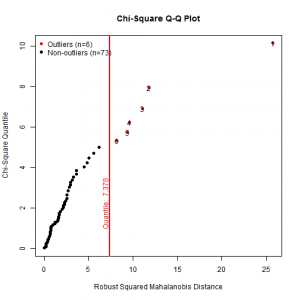Want to share your content on R-bloggers? click here if you have a blog, or here if you don't.In this set of exercises we will practice multivariate analysis of variance – MANOVA.

We shall try to find if there is a difference in the combination of export and bank reserves, depending on the status of banking sector (is there a crisis or not). The data set is fictitious and servers for education purposes only. It consist of variables `crisis`, which is factor, meaning that there exists or there does not exist banking crisis and `export` and `reserves`, in billions of currency units. You can download it here.

In this set of exercises we use two packages: `MVN` and `heplots`. If you haven’t already installed them, do it using the following code:

``` install.packages(c("MVN", "heplots")) ```

and load them into the session using the following code:

``` library("MVN") library("heplots") ```

before proceeding.

Answers to the exercises are available here.

If you have different solution, feel free to post it.

Exercise 1

Is the sample size large enough for conducting MANOVA? (Tip: You should have at least 2 cases for each cell.)

1. Yes
2. No

Exercise 2

Are there univariate and multivariate outliers?

1. There are univariate, but not multivariate outliers
2. There doesn’t exist a univariate outlier, but there are multivariate outliers
3. There exist both univariate and multivariate outliers

Exercise 3

How do you estimate univariate and multivariate normality of dependent variables?

1. Both variables are univariate normal, but they are not multivariate normally distributed
2. None of the variables is univariate normal, and hence there doesn’t exist multivariate normality
3. Both variables are univariate normal and the data is multivariate normally distributed

Exercise 4

Using the matrix of scatter plots, check for the linearity between dependent variables `export` and `reserves` for each category of independent variable.

Exercise 5

Calculate the correlation between dependent variables `export` and `reserves`. Is it appropriate to justify conducting MANOVA?

1. Yes
2. No

Exercise 6

Is there equality of covariances of the dependent variables `export` and `reserves` across the groups. (Tip: You should perform Box’s M test of equality of covariance matrices.)

1. Yes
2. No

Exercise 7

Is there equality of variances of the dependent variables `export` and `reserves` across groups? (Tip: Use Levens’s test of error variances.)

1. Yes
2. No

Exercise 8

On the level of significance of 0.05, is there effect of banking crisis to export and banking reserves combination?

1. Yes
2. No

Exercise 9

How much of the variance in the dependent variables `export` and `reserves` is explained by banking crisis?

Exercise 10

Does the export differ when banking sector is in the crisis compared to when banking sector is not in the crisis? What about reserves?

1. Only export differ
2. Only reserves differ
3. Both export and reserves differ
4. None of them differ